# How to Automatically Insert an Equal Sign in Cells with Numbers in Excel?

Let us assume a problem where we want to perform mathematical operations and you forgot to add the equal sign. In general, when solving operations, we must always keep an equal sign to treat it as a formula. This tutorial will help you understand how we can automatically insert the equal sign in cells with numbers in Excel. We will insert the equal sign and calculate the values automatically in this article.

Here we will first use the formula to insert the equal sign, then use the paste special, and finally use the find and replace function to complete our task. Let us see an uncomplicated process to see how we can automatically insert the equal sign in cells with numbers.

Step 1

Let us consider an Excel sheet where the data is like the data shown in the below image.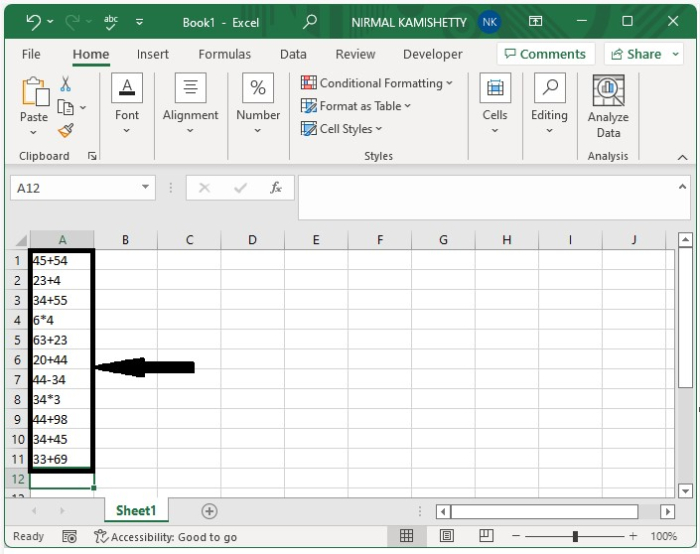Now click on the empty cell and enter the formula as "="&A1 to insert the equal sign at the beginning of every statement, as shown in the below image. In the formula, A1 is the cell where the data is present.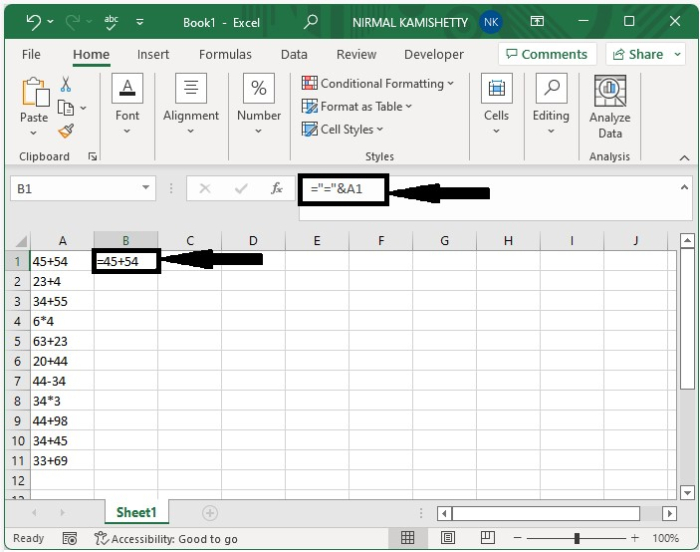Step 2

As we can see, the equal sign is inserted before the first cell. We can insert the equal sign into all the cells using the auto-fill handle, as shown in the below image.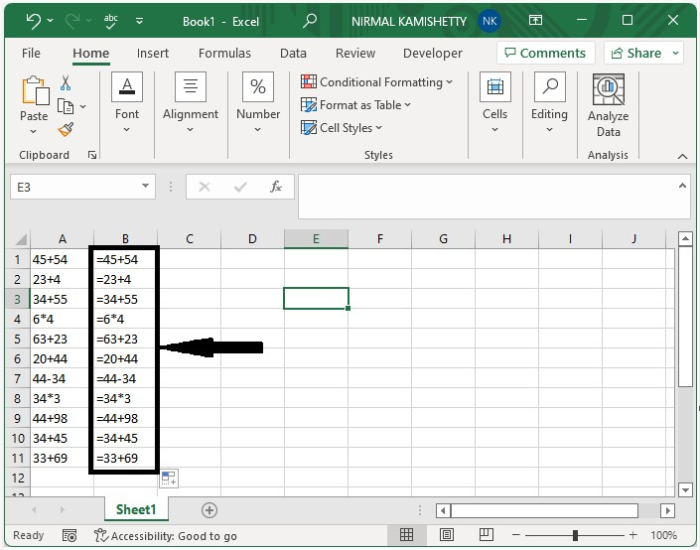Step 3

We have inserted the equal sign successfully. If you could have observed the statements, we can see that if we enter the statements, they will be executed.

Now select the data, then copy the cells using the command CTRL+C, click on paste under home, and click on paste values as shown in the below image.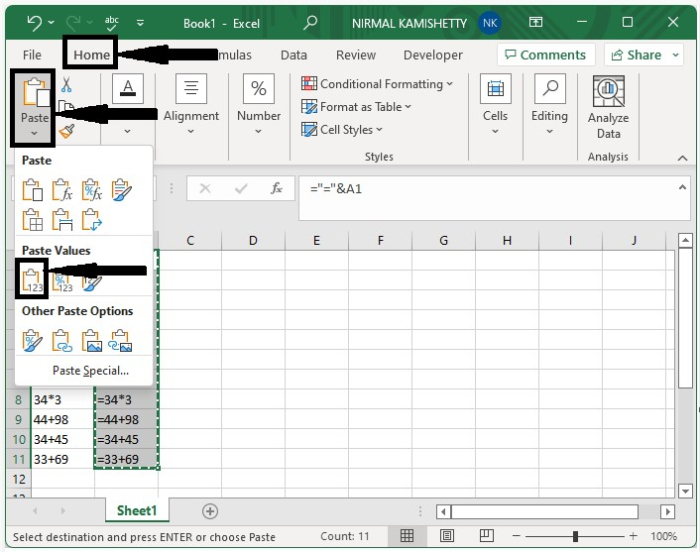Step 4

Now use the command CTRL+F to open find and replace, then enter = in find and = in replace, then click on replace all as shown in the below image.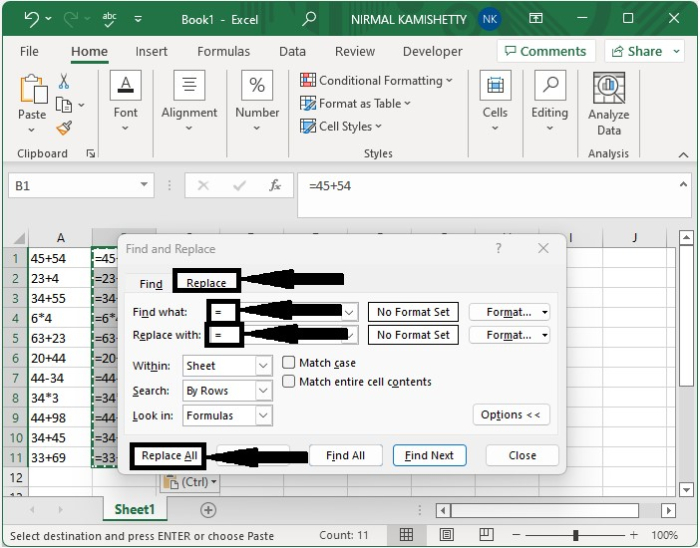Our end result will look like the image below.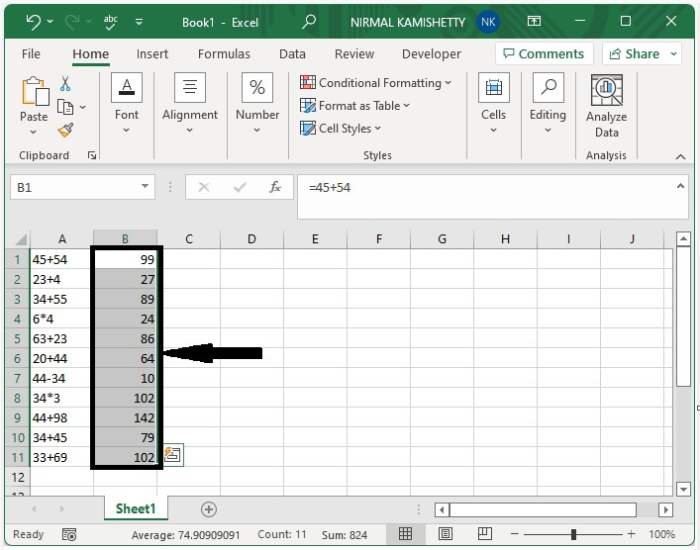## Conclusion

In this tutorial, we used a simple example to demonstrate how we can automatically insert equal signs in cells with numbers in Excel.

Updated on: 10-Jan-2023

4K+ Views